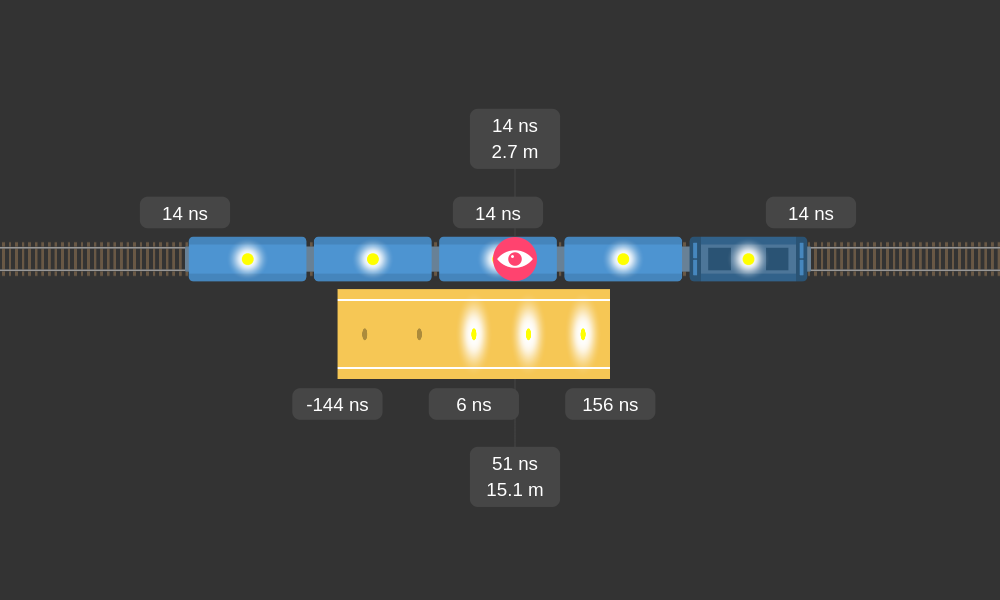This article is based on the Train-platform paradox simlation available at https://train.tdworakowski.com.

If you consider two relativistic phenomena which are length contraction and time dilation, the special theory of relativity may seem inconsistent. Imagine a train 100 meters long is passing a platform 100 meters long traveling at 90% of the speed of light. According to the theory, for the observer on the platform the train is shortened and the time inside it elapses more slowly. But for observer inside the train the length of the train is normal, time elapses normally, however the platform is shortened and the time on the platform elapses more slowly. How is it possible that both of these facts coexist?

To answer this question we need to understand the third relativistic phenomenon which is relativity of simultaneity. If we consider these three phenomena together, the theory becomes consistent. You can play with the simulation to confirm that the theory is consistent.

## Three phenomena

### Length contraction

An object which in its own inertial frame is $$L_0$$ in length, in the inertial frame in which is moving at velocity of $$V$$ is shorter being $$L$$ in length.

$L = L_0 \, \sqrt{1 - \left({V \over c}\right)^2}$

• $$c$$ - the speed of light
• the length of the object is measured along the velocity vector

### Time dilation

Whilst in the inertial frame in which the object is moving at velocity of $$V$$ elapses $$T_0$$ of time, in the own inertial frame of that object elapses less time $$= T$$.

$T = T_0 \, \sqrt{1 - \left({V \over c}\right)^2}$

• $$c$$ - the speed of light

### Relativity of simultaneity

Two clocks, which are synchronized in their own inertial frame, are not synchronized in the inertial frame, in which they are moving at velocity of $$V$$. The clock on the back shows the later value of time.

$T = T_0 + \left( -\Delta x \right) \, {V \over c^2}$

• $$c$$ - the speed of light
• $$\Delta x$$ - the position of the clock displaying time $$T$$ relative to the clock displaying time $$T_0$$ (the position is measured in their own inertial frame)

## The order of events

The order of two events may be different in different inertial frames. To show it using the simulation let's enable the Lights feature. When it is enabled all lapms (five on the train and five on the platform) will turn on at midnight sharp (time = 0 ns). Notice, that for different lamps (which are not in observer inertial frame) may be different local time.

Let's observe only two lamps: the front lamp on the train (on locomotive) and the first lamp from left on the platform.

When the observer is on the plafrom, first of the two observed lamps turns on the lamp on the plafrom.When the observer is in the train, first of the two observed lamps turns on the lamp on the train.